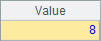• 集算器
教程
函数参考
习题
代码参考
用户参考
外部库使用指南
数据文件工具用户参考
DQL教程
集群管理器使用说明
SPL命令执行器用户参考
• 润乾报表
教程
填报教程
分析教程
报表中心教程
用户参考
程序员参考
高级指南
通用查询
DQL部署集成手册
• 易明建模
用户参考
json参数说明
• 润乾官网

# 使用序列

## 生成

 A B C 1 1 red 2013-06-04 2 2 blue 27.49 3 3 yellow Tom 4 [15.2,b,1] =[A1:C3] =[3,A4,B4] 5 [1,2,3,3] [] [[]]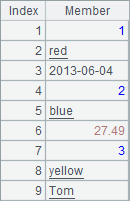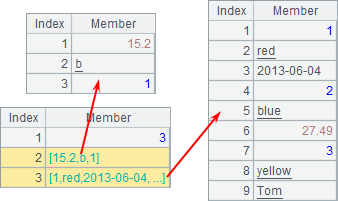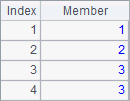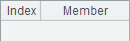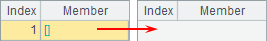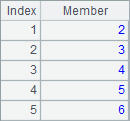A 1 =to(2,6) 2 ="1,a,b,c".split@c() 3 =periods@y("2014-08-10",date(now()),1) 4 =file("sales.txt").import@t()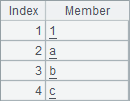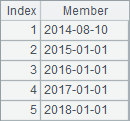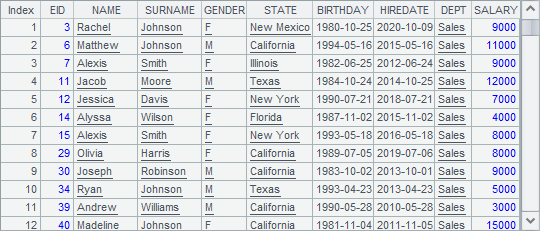A4从结构化的文本文件中读取记录形成序列，其值为：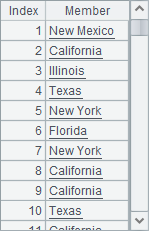A 1 =file("sales.txt").import@t() 2 =A1.(STATE) 3 =A1.group(STATE)A3中的序列如下：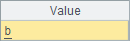## 访问

 A B 1 [a,b,c,d,e,f,g] 2 =A1(2) =A1.m(2) 3 =A1([2,3,4]) =A1(to(2,4)) 4 =A1.m(-1)

A2B2中的表达式是等价的，都是从序列中取出第2个成员，结果如下：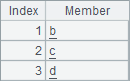A3中从序列中取出第24个成员，值为序列。注意，[2,3,4]也是个序列（数列），因此B3中中表达式的结果和A3中是相同的：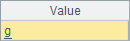A4中取出序列中的倒数第1个成员。注意，倒取数时必须用A.m()函数，不能简写为A1(-1)。结果如下：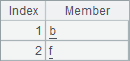A B 1 [a,b,c,d,e,f,g] 2 =A1.m(2,-2) =A1.m(2:4,3:5) 3 =A1.m(-1,4:-2,5) =A1.m(-2:2)

A2中获取序列中的第2个和倒数第2个成员，B2中获取序列中两段区间中的成员，A3中在获取序列成员时，同时使用了指定位置和区间的方式。A2,B2A3中的结果依次如下：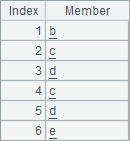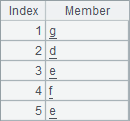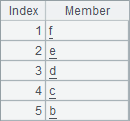A B 1 [a,b,c,d,e,f,g] 2 =A1.to(2,4) =A1.to(4,2) 3 =A1.to(3) =A1.to(-3)

A2,B2,A3,B3中的结果如下：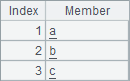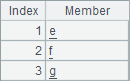B2的结果中可以看到，与A.m()不同，A.to()函数在指定区间时，可以由后至前，此时获得序列中成员的顺序也是逆序的。A3中表示获得序列的前3个成员，B3中表示获得序列的最后3个成员。

 A 1 [a,b,c,d,e,f,g] 2 >A1(2)="r" 3 >A1([3,4])=["r","s"] 4 >A1.modify(4,[ "r","s"])

A2A1中的第2个成员修改为rA3继续修改A1中的第3、第4个成员。A4从第4个成员起继续依次修改，该表达式等价于>A1([4,5])=["r","s"]，逐步运行时A1中序列的变化如下：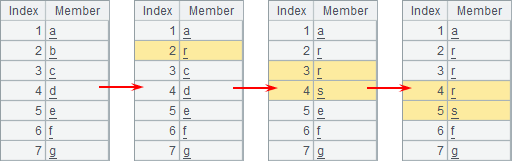A 1 [a,b,c,d,e,f,g] 2 >A1.insert(0,"r") 3 >A1.insert(2,["r","s","t"])

A2在序列结尾新增成员，A3继续在第2个成员之前连续插入3个新成员。仍然使用分步执行，A1中序列的变化如下：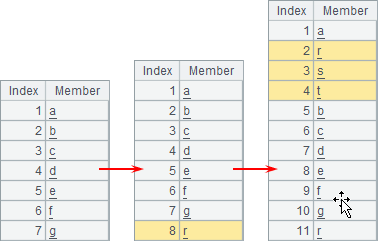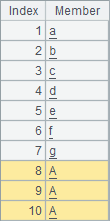A 1 [a,b,c,d,e,f,g] 2 > A1.delete(2) 3 > A1.delete([2,4])

A2中删除第2个成员，A3中继续删除第2、第4个成员，分布执行时，A1中变化如下：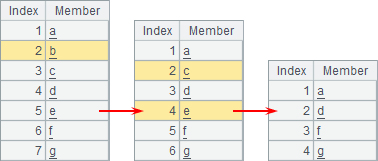## 运算符

 A B 1 [a,b,1,2,3,4] [d,b,10,12,3,4] 2 =A1^B1 =A1\B1 3 =A1&B1 =A1|B1

A1B1中的序列如下：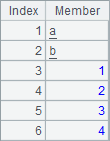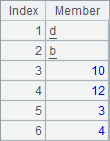A2,B2,A3,B3中，分别计算这两个序列的交集，差集，并集和合集。计算后，A2,B2,A3,B3中的结果分别如下：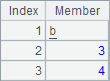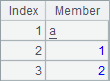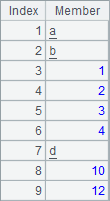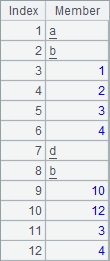A B C 1 [3.456,5L,,2,-3,4] =["d","b","Ace",,"Tom","3"] [a,b,1,2,3,4] 2 =A1.sort@z() =B1.sort() =C1.sort()

A1中序列的成员包括各种实数及空值，B1中的成员包括字符串或空值，A1B1中的成员均可以比较。A2B2中排序后的结果如下：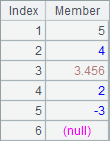C1中的序列中，既包括字符串，又包括整数，两种类型的数据是不能比较的，因此C2在计算时会报错：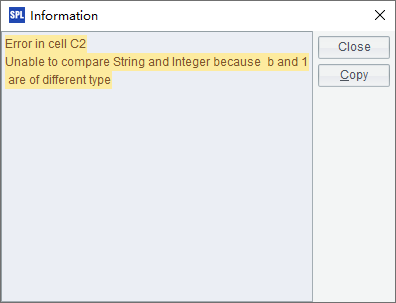A B 1 [1,2,3,4] [10,12,3,4] 2 =A1++B1 =A1--B1 3 =A1**B1 =A1//B1

A1B1中的序列如下：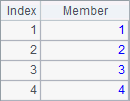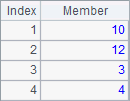A2,B2,A3,B3中，分别用这两个序列计算对位加法，对位减法，对位乘法和对位除法。计算后，A2,B2,A3,B3中的结果分别如下：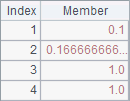Ø  cmp(A,B)

 A 1 =cmp(["a","b","c"],["a","b","c"]) 2 =cmp([1,3,5,7],[1,3,7,5]) 3 =cmp([7,6,5,4],[7,6,4,10,11])

A1,A2A3中结果如下：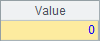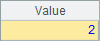A 1 =[1,2,3]==[1,2,3] 2 =[1,"B",3]<=[1,"b",4] 3 =[1,2,3]<[1,3,4]

A1中比较结果为true，两个序列相等。A2中比较结果为true，因为Bb小。A3中结果为true，因为两个序列的第2个成员比较时，23要小：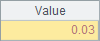A 1 [Tom,Jerry,Tuffe,Tyke] 2 [Jerry,Tuffe,Tom,Tyke] 3 =A1==A2 4 =A1.eq(A2)

A1A2中成员的顺序不同，因此A3中的结果表明两个序列不相等：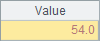A4中的结果为true，说明两个序列的成员相同：## 函数

 A 1 a,1,c,2011-8-11,false 2 =A1.split@c() 3 =A2.concat@c()

A2A3中结果如下：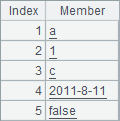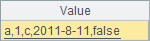regex函数，最简单的用法就是判定某个字符串与指定正则表达式的匹配结果，下面先来看一下与数字字符串的匹配用法：

 A B C 1 ="a12b".regex("(a[0-9])") ="a12b".regex("(a[0-9]*)") ="a12b".regex("[0-9]b") 2 ="a12b".regex("\\S*([0-9][a-z])") '\S*([0-9][a-z]) ="a12b".regex(B2)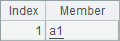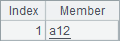A1中使用的正则表达式是"(a[0-9])"，其中a就是对应字母a本身，而[0-9]表示09之间的1个字符，即1位数字，两边的小括号()表示把字符串中能匹配“字母a开头后接1位数字”的串取出返回，结果就是单一成员a1构成的序列。B1[0-9]后面的*表示连续匹配前面的字符任意次，这里表示连续的任意位数字，因此B1中返回的结果是a12C1中，需要返回数字开头后面接着字母b的字符串，但是a12b并非是“以数字开头”的，因此无法匹配，C1中的结果是null

A2中使用的正则表达式是"\\S*([0-9][a-z])"，其中[a-z]表示字母az之间的字符，即所有小写字母。\S*表示连续的任意个可见字符，由于\是字符串中的转义字符，因此需要用\\S*表示。在这里，用小括号表示取出的部分是后面匹配[0-9][a-z]的结果，而前面匹配\S*的部分会被丢弃。在B2中，用字符串常数来表示A2中的正则表达式，此时不涉及字符串转义问题，所以C2中可以获得和A2中相同的结果。A2C2中的结果如下：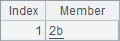A B C 1 ="a12b".regex@c("(A[0-9])") ="a12b".regex@c("([A-Z][0-9])") ="a12b".regex("([A-Z][0-9])")

A1,B1C1中结果如下：A1中正则表达式中要求匹配大写字母AB1中要求匹配任1个大写字母，由于使用了@c选项，因此用regex() 都能得到匹配结果a1，而C1中未使用@c选项，无法匹配正则表达式，返回null

 A B 1 ="Gerente de Fábrica".regex(".* (.*á.*)") ="Gerente de Fábrica".regex@u(".* (.*\\u00e1.*)")

A1的正则表达式.* (.*á.*)中，小数点 . 表示除回车符和换行符外的任一个单字字符，.*则表示任意个字符，A1中取出的是从最后一个包含字符á的单词之后的字符串。B1中的正则表达式是同样的意义，不过regex函数添加了@u选项，其中的字符áunicode的表示方法，表示为\u00e1regex@u() 通常用于解析外部字符串，通过unicode设置正则表达式可以避免不同字符集的干扰。A1B1中的结果是相同的：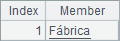A 1 [2,4,6] 2 =A1.sum() 3 =A1.sum(~*~)

A2对序列求和，A3求序列中成员的平方和，A2A3中的结果如下：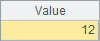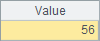A B C D 1 =[1,2,3,4].cand(24%~==0) =[12,-3,0].cor(24%~==0) [] 2 for 500 =A2%3==2 =A2%5==3 =A2%7==2 3 if [B2:D2].cand() >C1=C1|A2

A1中，判断序列[1,2,3,4]中的成员，是否全部是24的约数，B1中则判断[12,-3,0]中的成员是否包含了24的约数。中国古代有一道著名算题：“今有物不知其数，三三数之剩二；五五数之剩三；七七数之剩二。问物几何？”在第23行，计算了这道题目在500以内的解，记录在了C1中。计算后，A1,B1C1中结果分别如下：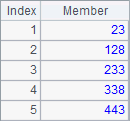A 1 [2,3,3,2,5,7,1] 2 =A1.count() 3 =A1.icount()

A2中计算序列中所有成员的个数，而A3中只计算不同成员的个数，A2A3中的结果如下：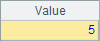A B C 1 [6,8,1,3,7,2,4,9,5] 2 =A1.rank(8) =A1.rank@z(8) 3 =A1.median() =A1.median(1:4) =A1.median(:3)

A2中计算8在序列中从小到大的排名，B2中则计算8在序列中从大到小的排名，A2B2中结果分别如下：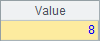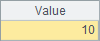A3计算序列A1中的中位数，即序列中值的大小位于最中间的一个成员，如果序列中成员为偶数个，那么中位数将返回最中间的两个成员的平均值。如果在median函数中添加参数，则可以取出序列中成员按照从小到大的顺序，处于参数所指定分段点的成员，类似的，如果分段点正好处于两个成员中间，则会返回两个成员的平均值。如B3中即返回位于1/4点的数据，因此，A.median(k:n)的参数中，分段数n必须为不小于2的整数，而k必须小于。当参数中的k省略时，将返回序列n等分点位置的数据。A3,B3C3中的结果如下：A B 1 [[1,2,3],,[3,4],[6,5,3]] 2 =A1.conj() =A1.union() 3 =A1.diff() =A1.isect()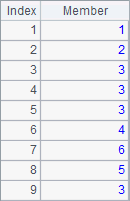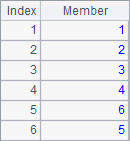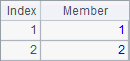A B C 1 [2,4,-6] =A1.(~+1) 2 =A1.select(~>1) =A1.pselect@a(~>1) =A1.pos([-6,2]) 3 =A1.ranks@z() =A1.sort() =A1.sort(-~)

B1将序列中每个成员加1，结果如下：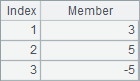A2过滤出大于1的成员，B2定位出大于1的所有成员的序号，C2查找成员-62在序列A1中的序号。A2,B2C2中的结果如下：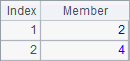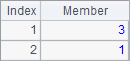A3中，通过在ranks函数中添加@z选项，按降序求得序列各成员的排名，B3将序列中的成员升序排序，C3则将序列中的成员降序排序。A3,B3C3中的结果如下：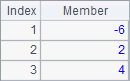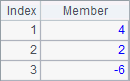A B 1 [2,4,-6,null,4,3,] 2 =A1.pselect@a(~>1, 5) =A1.pos(4,3)

A2中，从第5个成员开始查找大于1的成员位置，B2中从第3个成员开始查找4的位置，A1B2中结果如下：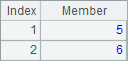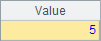A B C 1 [2,4,-6,null,4,3,] 2 =A1.pselect(~>10) =A1.pselect@0(~>10) =A1.pos(5) 3 =A1.pos@0(5) =A1.pmin() =A1.pmin@0()

A2,B2,C2,A3,B3,C3执行后的结果如下：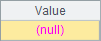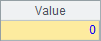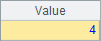A B 1 [2,4,-6,null,4,3,] 2 =A1.pselect@n(~>10) =A1.pos@n(5)

A2B2中的结果是相同的：